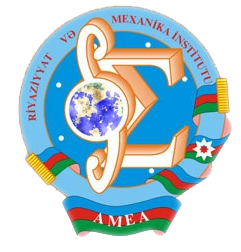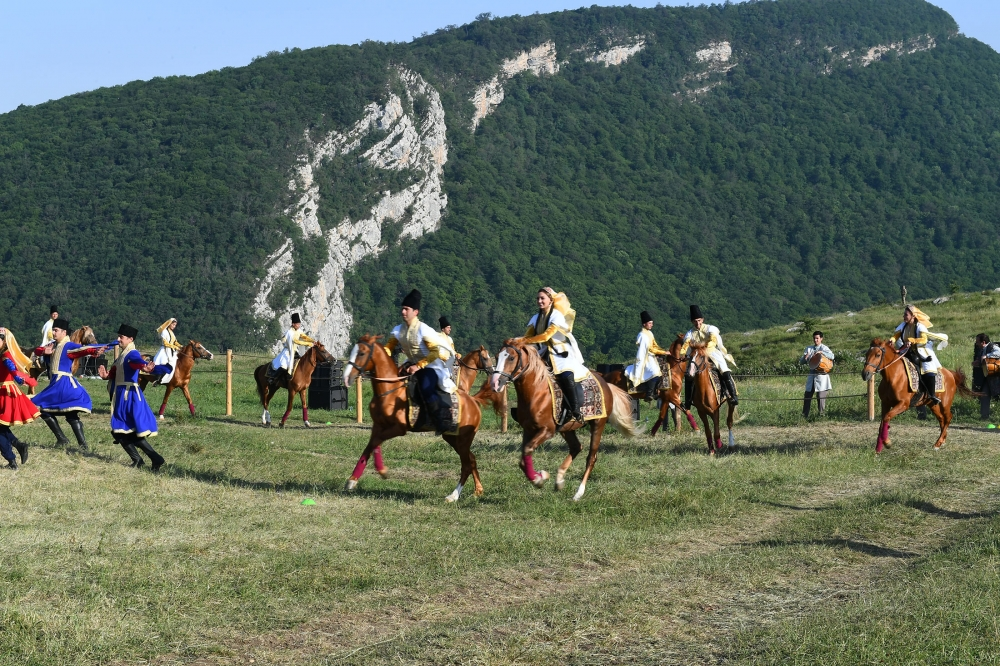Riyaziyyat və Mexanika İnstitutu

## Qarabağ xəbərləri### Şuşada Heydər Əliyev Fondunun təşkilatçılığı ilə “Musiqi irsi və Qarabağ atları Cıdır düzündə” adlı kompozisiya təqdim olunub## Transactions 2001/1/XXI

 Year: 2001 Volume: 1 Number: XXI About: [PDF] MATHEMATICS Abbasov N.Yu. Abstract [PDF] A theorem on the oscillation of solutions of nonuniformly degenerate parabolic equations of second order [PDF] 3 Akhundov A.Ya. Abstract [PDF] On one inverse problem for a semi-linear equation of parabolic type. [PDF] 13 Aliev A,B., Ahmedova Zh.B. Abstract [PDF] Global solutions of non-linear fourth order hyperbolic inequalities [PDF] 20 Aliguliyev R.M. Abstract [PDF] A Phragmen-Lindeloftype theorem for a non-divergent structure linear elliptic equation of the second order [PDF] 26 Amanov R.A. Abstract [PDF] The generalized solvability of the Dirichlet problem for non-uniformly degenerating elliptic equations of the second order [PDF] 32 Amirov R.Kh., Cakmak Y. Abstract [PDF] Trace formula for the Sturm-Liouville operator with singularity at x = 0 [PDF] 42 Guseinov R.V. Abstract [PDF] Theorem onfiniteness of negative spectrum ofquasielliptic operator. [PDF] 40 Aslanov G,I. Abstract [PDF] Partial operator-differential equations in spaces of type S [PDF] 49 Bairamov R.A. Abstract [PDF] On connections between function clones and varieties of algebras. [PDF] 56 Guliyev V.S., Mustafayev R.Ch. Abstract [PDF] On generalized fractional integrals. [PDF] 63 Ibikli E. Abstract [PDF] Approximation of Bore! derivatives of functions by singular integrals. [PDF] 72 Iskenderov N.Sh., Jabbarova K.A. Abstract [PDF] The scattering problem for a hyperbolic system of five first order equations on semi-axis with two given incident wave. [PDF] 77 Jafarov N.J. Abstract [PDF] Unique strong solvability of the first boundary value problem for parabolic Gilbarg-Serrin equation in the paraboloid type domains. [PDF] 83 Mamedov F.I. Abstract [PDF] Poincare type weight inequalities in domains with an isoperimeiric type condition. [PDF] 93 Mamedov I.T., Salmanova Sh.Yu. Abstract [PDF] The A.D. Aleksandrov type inequality for a class of second order equations with non-negative characteristic form. [PDF] 103 Najafov Kh. M. Abstract [PDF] On one general boundary value problem with displacement for one second order hyperbolic equation [PDF] 111 Salmanova Sh.Yu. Abstract [PDF] The apriori estimate of Holder’s norm of solutions of degenerate elliptico-parabolic equations of the second order [PDF] 117 Soltanov K.N., Fayziyev A.S. Abstract [PDF] On the complexity characteristics of some problems [PDF] 122 Suleymanov S.E., Mamedova Sh.D. Abstract [PDF] Limiting absorption principle for the Helmholtz equation in a many- dimensional layer with a general boundary condition [PDF] 129 Vahabov N.G. Abstract [PDF] Localization of spectrum and its applications. III Numerical range and spectrum of operator-functions [PDF] 138 MECHANICS Abbasova L.A. Abstract [PDF] Propagation of the failure front in the damaged round thick pipe subjected to temperature [PDF] 147 Amiraslanov I.A., Abdullayeva L.A., Amiraslanova N.I. Abstract [PDF] Development of canal in a packed layer [PDF] 155 Kazimova R.A. Abstract [PDF] Torsion of visco-elastic prismatic pivots in geometric and physical non-linear statement [PDF] 159 Mutallimov Sh.M. Abstract [PDF] On normal impact on flexible elastic filament [PDF] 165 Rzayev O.G. Abstract [PDF] Buckling around the two collinear macro-cracks in the clamped composite plate-strip [PDF] 173 Talybly L.Kh. Abstract [PDF] On the equation of fatigue wear at external friction taking into account the influence of loading history [PDF] 179 Veliev A.A.. Abstract [PDF] The approximation of solution of the stationary problem of saline fingers at high supercriticality [PDF] 186 Yusifov M.O. Abstract [PDF] On non-linear longitudinal vibration of rectilinear pile [PDF] 194 APPLIED PROBLEMS OF MATHEMATICS AND MECHANICS Gasanov K.K., Guseynova Kh.T. Abstract [PDF] The necessary conditions of optimality in the problem for one class systems of partial differential equations of the first order with ordinary and generalized controls [PDF] 197 Guliyev I.K. Abstract [PDF] On fatigue wear of elastico-plastic plate at pulsing temperature action [PDF] 209 Kurbanov I.A., Mekhtiyeva G.Yu. Abstract [PDF] On two approximated methods for solution of one boundary value problem for a differential equation of the fourth order [PDF] 216 Melikov A.Z., Fattahova M.I. Abstract [PDF] Performance analysis and optimization of buffer allocation strategies: a state space merging approach [PDF] 222 Tagiyev R.K. Abstract [PDF] On the optimal control problem by coefficients of a hyperbolic equation [PDF] 230
Azərbaycanda COVID-19 ilə bağlı statistika
• Virusa yoluxan

599713

• Sağalan

569238

• Yeni yoluxan

1210

• Aktiv xəstə

22456

• Ölüm halı

8019

• Test edilib

5,633,054